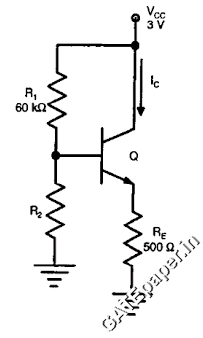### GATE 2013 ECE Video Solutions on EDC (Electronic Devices and Circuits)

1. In IC technology, Dry oxidation(using dry oxygen) as compared to Wet oxidation (using stream or water vapor) produces………..
a. Superior quality oxide with a higher growth rate
b. Inferior quality oxide with a higher growth rate
c. Inferior quality oxide with a lower growth rate
d. Superior quality oxide with a lower growth rate

2. For a forward biased PN junction diode, the sequence of events that best describes the mechanism of current flow is
a. Injection and subsequent diffusion and recombination of minority carriers
b. Injection and subsequent drift and generation of minority carriers
c. Extraction and subsequent diffusion and generation of minority carriers
d. Extraction and subsequent drift and recombination of minority carriers

3. In MOSFET operating in saturation region, the channel length modulation effect causes
a. An increase in gate source capacitance
b. A decrease in Transconductance
c. A decrease in unity gain bandwidth product
d. A decrease in output resistance

4. In the circuit shown below, the knee current of ideal zener diode is 10 mA. To maintain 5 volts across the load RL, the minimum value of RL in Ω’s and the minimum power rating of the zener diode in mW respectively area. 125 and 125
b. 125 and 250
c. 250 and 125
d. 250 and 250

5. In the circuit shown below, the silicon NPN transistor Q has a very high value of β. The required value of R2 in KΩ to produce IC = 1 mA isa. 20
b. 30
c. 40
d. 50